# Starting up¶

## What is Jupyter?¶

Jupyter is a notebook interface for programming, and presenting information. This document is actually a Jupyter notebook. Many programming languages work with Jupyter, but we will be using Python 3. You can read more about Jupyter on the jupyter website.

There are many ways to run Jupyter. You can install python locally on your computer. Depending on how it is installed, python may include access to the jupyter notebook, or you may need to install it:

Jupyter allows for text to be written in Markdown and LaTeX. Markdown makes it easy to write web documents with simple syntax. This Markdown cheatsheet makes it easy.

In particular, LaTeX allows the inclussion of formulas, e.g., $$\frac{n(n+1)}{2}=\sum_{k=1}^n k.$$

## Microsoft Azure Notebooks¶

Microsoft Azure Notebooks is a free service allowing you to run Jupyter notebooks. This allows you to run Jupyter and Python without installing it on your own machine.

City College students already have a Microsoft account coming from the college. So you can use this account to log in. But, you could also use a personal account if you prefer. (There seems to be no benefit to using your college login. I assume Azure Notebooks was not part of the Education deal.)

## Creating a project.¶

Once you set up your account, you should be able to create a project. Click the button: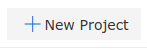A form will come up. You might fill it out something like this: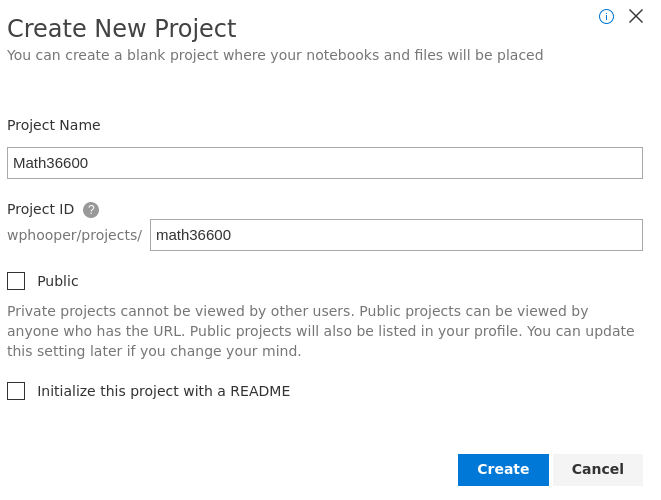Then click "Create". You should be brought to an empty project page looking like the following screenshot.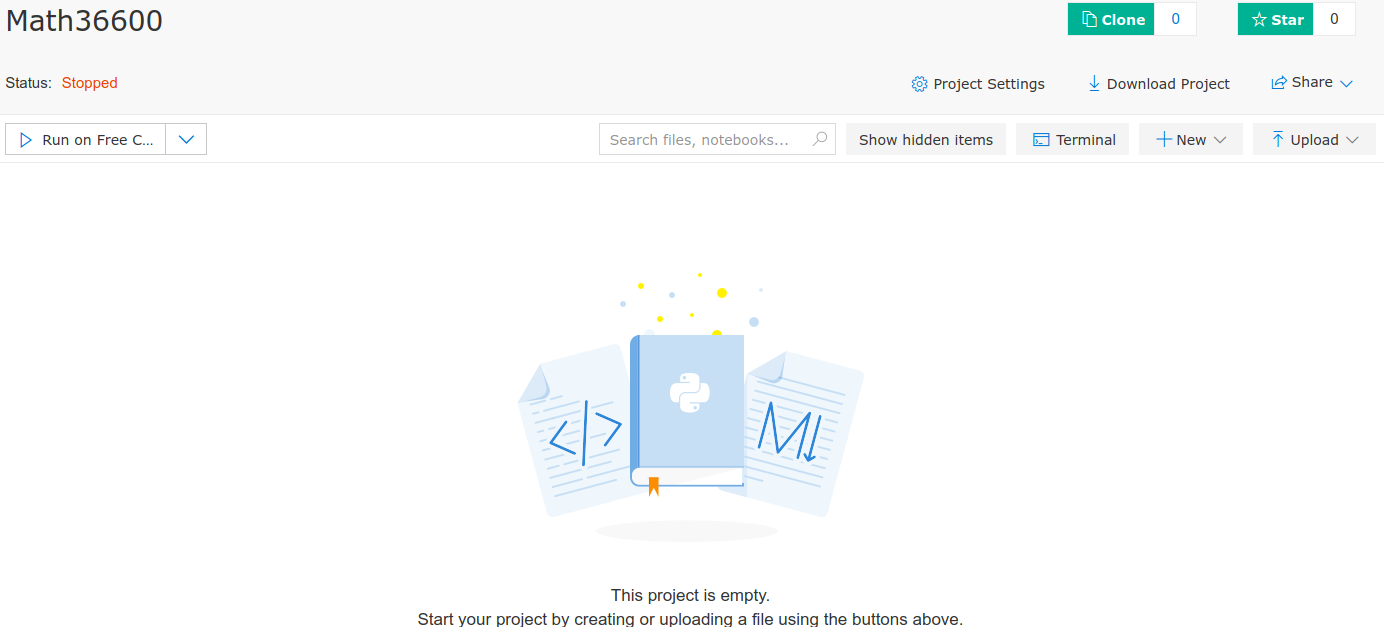## Create a Jupyter Notebook¶

To create a notebook, click the "+New" button. Then select "Notebook" to create a new Jupyter notebook. I dialog should appear. Enter "HelloWorld" for the name of the notebook. The ".ipynb" file extension should be appended automatically. This is the standard Jupter notebook file extension. Select "Python 3.6" for the language (or whatever the most recent version of Python 3 is.) Your dialog should look like the following: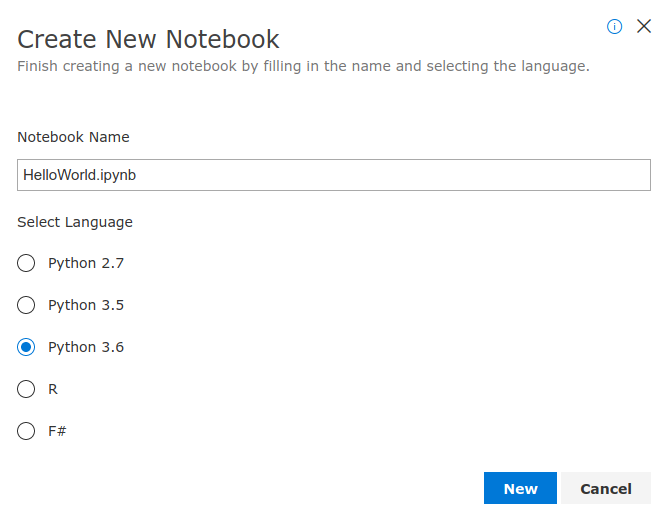Click "New" to create the notebook. Your view will switch from saying "This project is empty" to a file list containing the one notebook. Your project should now have a file list containing the one file:Click the file "HelloWorld.ipynb" to open the notebook.

## Run the Hello World program¶

With the notebook, open click on the first input cell. Then type print("Hello World!"). When you finish typing hold down SHIFT and press RETURN. You should see output similar to the following:

In :
print("Hello World!")

Hello World!


## Python as a calculator:¶

You can use Python as a calculator to get comfortable working with numbers in Python. Here are some examples.

In :
5+3

Out:
8
In :
35 * 45

Out:
1575
In :
1 / 3

Out:
0.3333333333333333
In :
2 * (3 + 4)

Out:
14

Exponentiation is denoted with the ** operator. (The symbol ^ is reserved for bitwise exclusive or.)

In :
2**3

Out:
8
In :
2^3

Out:
1

The number 52 divided by 3 is 17 with a remainder of 1. Python can compute 17 and 1 with the // and % operators.

In :
52 // 3

Out:
17
In :
52 % 3

Out:
1

Note that a // b always returns the greatest integer less than or equal to $\frac{a}{b}$. This is important for how answers are returned for negative numbers. Also if a // b is $c$ and a % b is $d$, then we have $a = b*c + d$. This explains the following output:

In :
-52 // 3

Out:
-18
In :
-52 % 3

Out:
2
In :
-52 // -3

Out:
17
In :
-52 % -3

Out:
-1

You can also test compare numbers with ==, !=, <, >, <= and >=. These represent the mathematical relations $=$, $\neq$, $<$, $>$, $\leq$ and $\geq$ respectively.

In :
3 == 5

Out:
False
In :
2**3 == 8

Out:
True
In :
2 < 3

Out:
True
In :
4 <= 3

Out:
False
In :
1 != 2 / 2

Out:
False
In :
1/3 == 0.3333

Out:
False
In :
1/3 == 0.333333333333333333333333

Out:
True

## Using the math library¶

The math library contains numerous useful mathematical functions, which are listed in the Python documentation for the math library.

Trigonometry is done in radians. For example, to compute $\cos \frac{\pi}{4}$, you might do the following:

In :
from math import pi, cos
cos(pi/4)

Out:
0.7071067811865476

The line from math import pi, cos allows us to use the pi constant and the cos function from the library. Once this is done, we can continue to use these in the rest of the file.

In :
cos(-2*pi/6)

Out:
0.5000000000000001

Note that we didn't import the sin function so typing sin(pi/6) would result in an error as depicted below: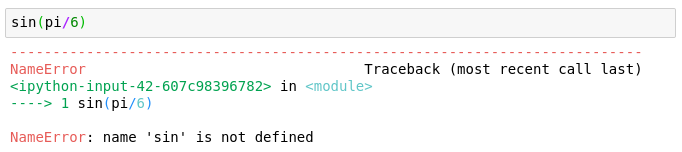We can solve this problem by importing sin as well.

In :
from math import sin
sin(pi/6)

Out:
0.49999999999999994

## Other types of importing¶

You can import a whole library, as demonstrated below.

In :
import math


When you import the whole library math as above you need to write math.something to access the something in the math library. For example

In :
math.tan(math.pi/4)

Out:
0.9999999999999999
In :
math.e

Out:
2.718281828459045

A good thing about doing this is you can learn about the math library by playing around. For example typing math. followed by pressing the Tab button will lead to a list of objects in the math library. When I do this, I get the following popup list.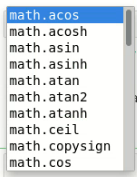See if you can also get this list to appear, and scroll through it.

Python also has self contained documentation. For example, you might wonder what the atan2 function does. Typing math.atan2? and pressing Shift+Return produces the documentation. Try it.

In :
math.atan2?


You can also see the documentation of things imported in the other way with something like cos?.

You can also import math with a different name. This is often used to simplify a library name or to avoid name clashes. For example the following imports the math library with the name m and does a simple calculation.

In :
import math as m
m.exp(2)

Out:
7.38905609893065

For more information on importing see §1.4 of Programming for Computations - Python by Svein Linge and Hans Petter Langtangen, 2nd edition.

## Including paragraphs¶

Jupyter supports including documentation blocks in addition to code blocks. Documentation can be written in a combination of Markdown, HTML, and LaTeX.

To create a documentation block first click on a new input box. Then switch from code to Markdown in the dropdown menu in the Jupyter toolbar.

Type some text, then press SHIFT+RETURN. The text should become part of the page. You can later edit the text by double clicking on it.

Markdown is a simple and easy to read markup that translates to HTML. You can learn more by reading this Getting Started Guide and this cheatsheet.

One useful markdown trick to know is how to create headings. You create them by creating a line that looks like: # Heading or ## Subheading. The symbol # must appear at the beginning of a line.

## Including mathematics¶

Markdown also supports LaTeX for documenting mathematics. Dollar signs are used on both sides of a LaTeX expression. For example typing $\frac{x+y}{2} \geq z$ yields $\frac{x+y}{2} \geq z$.

For more complicated expressions that should not be included in a paragraph, you use double dollar signs on each side to produce a centered equation. For example, $$\sum_{k=1}^n k = \frac{n(n+1)}{2}.$$ produces $$\sum_{k=1}^n k = \frac{n(n+1)}{2}.$$

The document LaTeX Math for Undergrads gives a lot of expressions that can be combined to form anything you'd like to do in the course. Try to experiment with LaTeX formulas.

You can also learn some LaTeX by looking at these Jupyter notebooks. If you see a LaTeX expression you want to learn, download the notebook, then upload it to Azure Notebooks. Then you can double click on the expression to see how it was typeset.